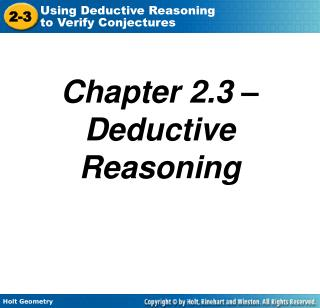DownloadDownload PresentationChapter 2.3 – Deductive Reasoning

# Chapter 2.3 – Deductive Reasoning

Download Presentation## Chapter 2.3 – Deductive Reasoning

- - - - - - - - - - - - - - - - - - - - - - - - - - - E N D - - - - - - - - - - - - - - - - - - - - - - - - - - -
##### Presentation Transcript

1. Chapter 2.3 – Deductive Reasoning

2. EQ Through logical thinking, how can I use the Law of Detachment and the Law of Syllogism?

3. Deductive reasoning Based on Logical reasoning. *facts, definitions, Properties etc. Inductive reasoning Based on patterns of specific cases.

4. Example 1A: Media Application Is the conclusion a result of inductive or deductive reasoning? There is a myth that you can balance an egg on its end only on the spring equinox. A person was able to balance an egg on July 8, September 21, and December 19. Therefore this myth is false. Inductive Reasoning

5. Example 1B: Media Application Is the conclusion a result of inductive or deductive reasoning? There is a myth that the Great Wall of China is the only man-made object visible from the Moon. The Great Wall is barely visible in photographs taken from 180 miles above Earth. The Moon is about 237,000 miles from Earth. Therefore, the myth cannot be true. Deductive Reasoning

6. Check It Out! Example 1 There is a myth that an eelskin wallet will demagnetize credit cards because the skin of the electric eels used to make the wallet holds an electric charge. However, eelskin products are not made from electric eels. Therefore, the myth cannot be true. Is this conclusion a result of inductive or deductive reasoning? Deductive Reasoning

7. Law of Detachment If _____ is a true statement and _p_ is true, then _q_ is true. p q

8. Example 2B: Verifying Conjectures by Using the Law of Detachment Determine if the conjecture is valid by the Law of Detachment. Given: In the World Series, if a team wins four games, then the team wins the series. The Anaheim Angels won four games in the 2002 World Series. Conjecture: The Angels won the 2002 World Series.

9. Example 2B: Verifying Conjectures by Using the Law of Detachment Continued Identify the hypothesisand conclusionin the given conditional. In the World Series, if a team wins four games, then the team wins the series. Is the statement true? _Yes_____ Is the hypothesis true? __Yes____ Is the conclusion true? __Yes____ So the conjecture is ___True________.

10. Check It Out! Example 2 Determine if the conjecture is valid by the Law of Detachment. Given: If a student passes his classes, the student is eligible to play sports. Ramon passed his classes. Conjecture: Ramon is eligible to play sports.

11. Check It Out! Example 2 Continued Identify the hypothesis and conclusionin the given conditional. If a student passes his classes, then the student is eligible to play sports. Is the statement true? __Yes____ Is the hypothesis true? __Yes____ Is the conclusion true? __Yes____ So the conjecture is ___True________.

12. Law of Syllogism If p q and q r are true statements, then p r is a true statement.

13. Example 3A: Verifying Conjectures by Using the Law of Syllogism Determine if the conjecture is valid by the Law of Syllogism. Given: If a figure is a kite, then it is a quadrilateral. If a figure is a quadrilateral, then it is a polygon. Conjecture: If a figure is a kite, then it is a polygon.

14. Example 3A: Verifying Conjectures by Using the Law of Syllogism Continued Let p, q, and r represent the following. • p: A figure is a kite q: A figure is a quadrilateral • r: A figure is a polygon. • You are given that p q and q r Is p q true? ________ Is q r true? ________ So the Conjecture is ________.

15. Example 3B: Verifying Conjectures by Using the Law of Syllogism Determine if the conjecture is valid by the Law of Syllogism. Given: If it is hot, then it is summer. If it is summer, then we are out of school. Conjecture: If it is hot, then we are out of school.

16. Example 3B: Verifying Conjectures by Using the Law of Syllogism Continued Let p, q, and r represent the following. • p: It is hot q: It is summer • r: We are out of school • You are given that p q and q r Is p q true? ________ Is q r true? ________ So the Conjecture is ________.